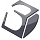## Beizer scaling the input

Post questions, comments and feedback to our 3Dconnexion UNIX and Linux Development Team.

Moderator: Moderators

Absum
Posts: 53
Joined: Tue Apr 24, 2007 4:00 am
Location: Sweden
Contact:

### Beizer scaling the input

I need some math help. I want to write something to scale the input from my spacenavigator. Preferably using a beizer curve with two control points. The problem is that I have no idea how to achieve this using c. If anyone could help me or point me in the right direction i would be really grateful.

crobl
ModeratorPosts: 131
Joined: Mon Feb 26, 2007 8:34 am
Location: Freiham, Germany
Hi Absum,

I must admit, I'm not really familiar with Bézier curves! For that reason I neither claim nor guarantee to be correct with my post!

From this article my idea for a quick shot would be to use two quadratic Bézier curves. This might be sufficient to scale the input, from what you write. Although I'd reconsider this idea depending on the quality (and correctness)As said, its just a quick idea:
Curve1 { C1P0(-1.0, -1.0), C1P1(x1,y1), C1P2(0,0) }
Curve2 { C2P0(0.0, 0.0), C2P1(x2,y2), C2P2(1.0,1.0) }

Where C1P1 and C2P1 are your two variable control Points.

Drawback:
The two functions will together behave somewhat discontinuous at the origin 0/0 for some values of C1P1 and C2P1, but you could probably attenuate this effect by ensuring, that the control points stay in their quadrants.
C1P1 should not leave the area enclosed by y1=m1*x and y2=m2*x, where m1<=1.0 and m2>=0, x<0. For C2P1 this would be the same, except: x>0.

You just need to calculate (with) one of the two curves, depending on the input value >0 or <0.

Now that t is element of [0,1], I'd use for the value mapping an increment tInc to be 1.0/512.0, where 512 is the theoretical maximum/minimum our device's axis can have.

To scale a device value I'd do:

Code: Select all

``````t = abs(deviceValue) * tInc;

if (deviceValue < 0)
{
scale = pow((1 - t), 2) * C1P0.y  + 2 * t * (1 - t) * C1P1.y + pow(t, 2) * C1P2.y;
}
else if (deviceValue > 0)
{
scale = pow((1 - t), 2) * C2P0.y  + 2 * t * (1 - t) * C2P1.y + pow(t, 2) * C2P2.y;
}
else
{
scale = 0.0;
}
outValue = deviceValue * scale;
``````

For drawing a curve:

Code: Select all

``````for (t = 0.0; t <= 1.0; t += tInc)
{
Presult.x = pow((1 - t), 2) * C1P0.x  + 2 * t * (1 - t) * C1P1.x + pow(t, 2) * C1P2.x;
Presult.y = pow((1 - t), 2) * C1P0.y  + 2 * t * (1 - t) * C1P1.y + pow(t, 2) * C1P2.y;
#ifdef LINE
drawALine(Pold, Presult);
Pold = Presult;
#else
drawPoint(Presult);
#endif
}
``````
I hope my idea is not too wrong and not too weird and I could help you with this.

Regards,

Christian
3Dconnexion

Absum
Posts: 53
Joined: Tue Apr 24, 2007 4:00 am
Location: Sweden
Contact:
Damn, i feel abit ashamed that I actually missed wikipedia this time :O

And thanks for the post I'll try this as soon as possible